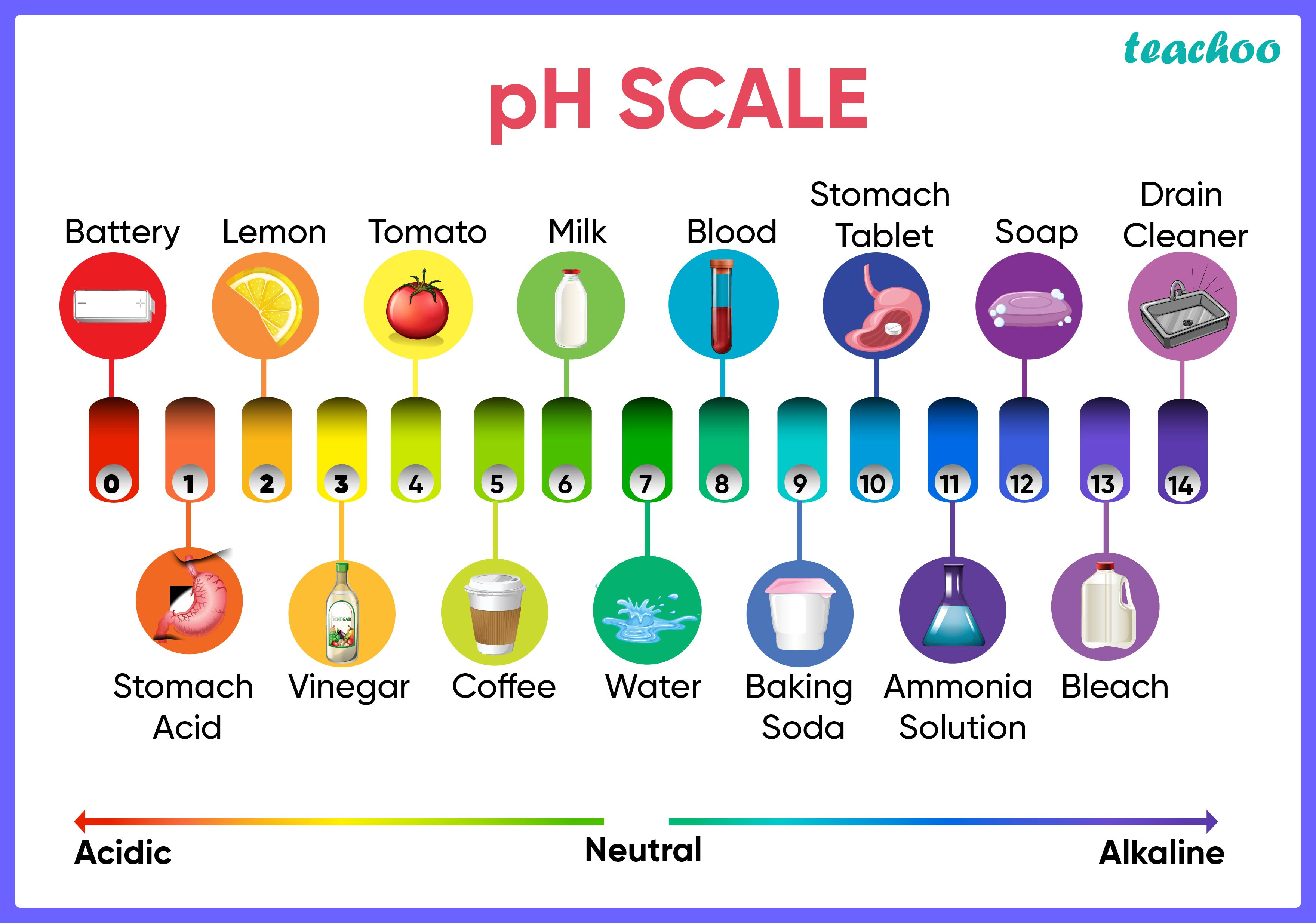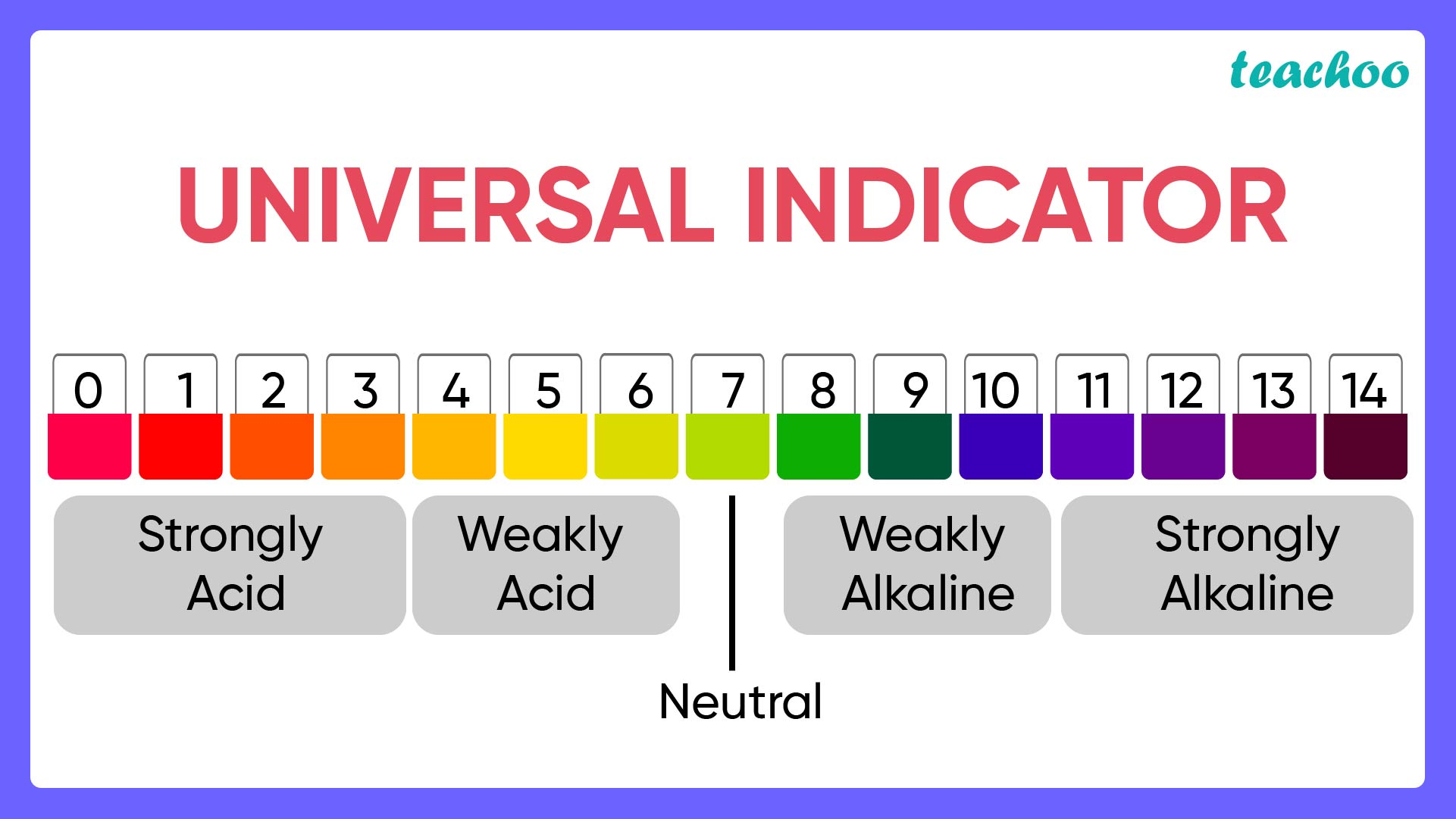## pH Scale

• pH is a value that shows how acidic or basic a substance is.
• The strength of an acid or base is measured on a scale of numbers from 0-14  called the pH scale.
• Due to water mixed with the acid or base , solutions of both acid as well as base will certainly contain hydrogen molecules. Except acids will have more hydrogen ions as compared to bases.
• The strength of an acid or base is nothing but the measure of these hydrogen ions present in their solutions.## Deciphering the pH value :

1. Acidic solutions will have a pH less than 7.
2. More Acidic a solution , lower will be its pH and thus stronger will be the acid.
3. Basic Solutions will have a pH of more than 7
4. More basic a solution , higher will be its pH and thus stronger will be the base.
5. As we move from 0 to 14 in the pH scale , the concentration of Hydrogen(H + ) ions reduces and the concentration of Hydroxide (OH - ) ions increases.

## Universal Indicator

The Universal Indicator is a mixture of many different indicators (or dyes) that gives different colours at different pH values of the entire pH scale. So looking at the colour obtained on the universal indicator paper , one can find out the pH of a given substance.Intext Question - Page 28 Q1

NCERT Question - Q1, Q9

1. Class 10
2. Chapter 2 Class 10 - Acids, Bases and Salts (Term 1)
3. Concepts